getDGM

Convert gain and phase variation into disk-based gain variation

Description

In disk margin analysis, gain and phase variations are modeled as a factor F(s) multiplying the open loop response L(s). This factor takes values in a disk D centered on the real axis with real-axis intercepts gmin and gmax. The disk margin determines the largest disk size [gmin,gmax] for which the feedback loop remains stable. This provides a gain margin of at least DGM = [gmin,gmax] and also some phase margin DPM determined by the disk geometry.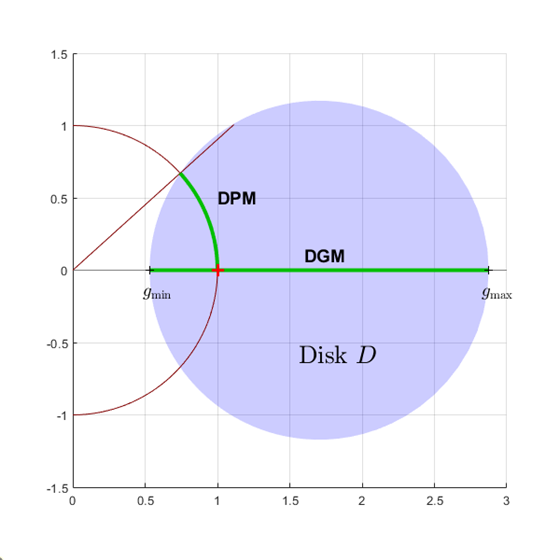Conversely, getDGM takes desired gain and phase margins GM and PM and computes the smallest disk D that delivers both. This disk is characterized by its real-axis intercepts gmin, gmax and the corresponding disk-based gain margin DGM = [gmin,gmax] and phase margin DPM meet or exceed GM and PM.

example

DGM = getDGM(GM,PM,'tight') computes the smallest disk that captures the target gain and phase variations specified by GM and PM.

• If GM and PM are scalars, then the disk captures gain that can increase or decrease by a factor of GM, and phase that can increase or decrease by PM.

• If GM and PM are vectors of the form [glo,ghi] and [pmin,pmax] then the disk captures relative gain and phase variations in these ranges.

• If either GM or PM is [], that removes the corresponding constraint on the disk size.

The output is of the form DGM = [gmin,gmax], and describes a disk that represents absolute gain variations within that range. For instance, DGM = [0.8,1.8] models gain that can vary from 0.8 times the nominal value to 1.8 times the nominal value, and phase variations determined by the disk geometry. This disk might have non-zero skew (see Algorithms). Use DGM to create a umargin block that models these gain and phase variations.

example

DGM = getDGM(GM,PM,'balanced') computes the smallest disk that represents a symmetric gain variation, that is, DGM = [gmin,gmax] where gmin = 1/gmax. This disk has zero skew (see Algorithms).

example

[DGM,DPM] = getDGM(___) also returns the disk-based phase range DPM modeled by the disk that DGM describes. You can use this output argument with any of the previous syntaxes.

Examples

collapse all

Find the smallest disk-based gain margin that represents relative a gain variation of ±6 dB relative to the nominal value and phase variation of ±40°. Convert the gain variation into absolute units.

GM = db2mag(6)
GM = 1.9953
PM = 40;
DGM = getDGM(GM,PM,'tight')
DGM = 1×2

0.4299    1.9953

DGM describes a disk that models both gain and phase variations. The values in DGM represent the range of gain variation in the absence of phase variation. Note that the DGM range is slightly larger than the specified [1/GM,GM] range as the phase margin requirement is more stringent and determines the disk size. Visualize the full range of gain and phase variations represented by DGM.

diskmarginplot(DGM)The 'tight' constraint computes the smallest disk that delivers both target gain and phase variations, which does not necessarily represent a symmetric gain range. In this case, the disk represents gain that can decrease somewhat more than it can increase. Examine the disk of uncertainty defined by this particular DGM.

diskmarginplot(DGM,'disk')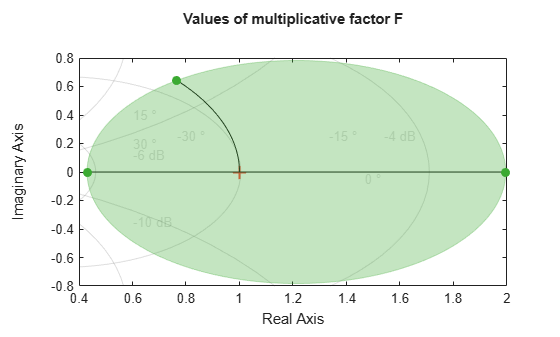To enforce symmetric gain variation, use the 'balanced' option.

Determine the disk-based gain margin that delivers symmetric gain variation of $±$5 dB and phase variation of $±$30 degrees.

GM = db2mag(5);
PM = 30;
DGM = getDGM(GM,PM,'balanced')
DGM = 1×2

0.5623    1.7783

The 'balanced' constraint models a disk of uncertainty that is symmetric around the nominal value. The function returns a symmetric disk-based gain margin DGM = [gmin,gmax], with gmin=1/gmax.

diskmarginplot(DGM)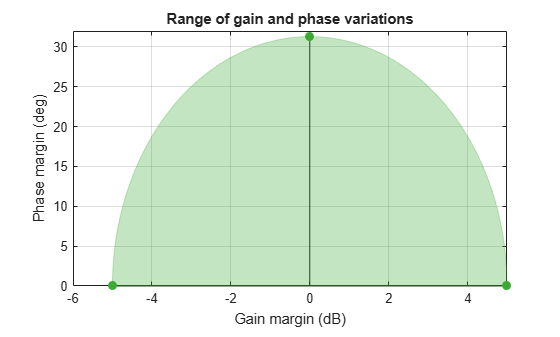In this case, DPM slightly exceeds the target phase variation and DGM is equal to the target gain variation.

Determine the disk-based gain margin corresponding to gain variations between 90% and 160% of the nominal value, and phase variations from -15 to +15 degrees.

gainRange = [0.9,1.6];
phaseRange = [-15,15];
DGMt = getDGM(gainRange,phaseRange,'tight')
DGMt = 1×2

0.8603    1.6000

The 'tight' constraint models the smallest disk that delivers target gain and phase variations. This disk is modeled with gain variation that skews toward gain increase.

Alternatively, you can use the 'balanced' option to constrain the disk-based gain margin to a symmetrical range of the form gmin = 1/gmax. This means that the gain can increase or decrease by equal amount.

DGMb = getDGM(gainRange,phaseRange,'balanced')
DGMb = 1×2

0.6250    1.6000

Visualize the range of simultaneous gain and phase variations corresponding to both gain ranges.

diskmarginplot([DGMt;DGMb])The balanced range DGMb models a larger, symmetric gain range (gmin = 1/gmax) and larger phase variations than the ones you specify. If you are confident that gain varies more in one direction than the other in your system, then this balanced model might be overly conservative.

Determine the balanced disk-based gain margin ranges that delivers gain variations of ±4 dB, ±6 dB, and ±12 dB and phase variation of ±30°. You can get all the disk-based gain ranges at once by stacking the desired target ranges into a column vector.

GM = db2mag([4;6;12]);
PM = 30;
DGM = getDGM(GM,PM,'balanced')
DGM = 3×2

0.5774    1.7321
0.5012    1.9953
0.2512    3.9811

diskmarginplot(DGM)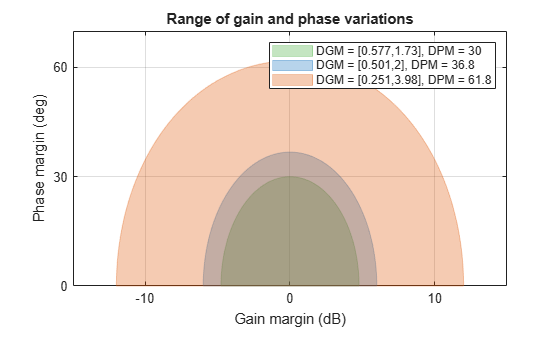Each row in the matrix DGM gives the disk-based gain variation for the corresponding entry in GM. For instance, the smallest balanced (symmetric) disk that captures gain variation of ±4 dB and phase variation of ±30° is specified by DGM(1,:) = [0.58 1.73].

This disk represents somewhat more than the target ±4 dB, in order to capture the full target gain variation of ±30°. For the targets ±6 dB and ±12 dB, the disk meets the target gain variation exactly, but the corresponding disks describe larger phase variations.

Input Arguments

collapse all

Target range of relative gain variation, specified as a scalar, vector, or two-column matrix.

• If GM is a scalar, then the disk captures gain that can increase or decrease by a factor of GM. For instance, if GM = 2, then the output DGM represents gain that can decrease or increase by a factor of 2.

• If GM is a vectors of the form [glo,ghi] then the disk captures relative gain variations in this range. For instance, if GM = [0.8,1.9], then DGM represents gain that can vary between 0.8 and 1.9 times the nominal value.

• If GM [], then getDGM returns a disk that captures the phase variation specified by PM, and the corresponding gain variation determined by the disk model.

Multiple Ranges at Once

To get DGM corresponding to multiple target gain ranges at once, specify GM as a column vector [GM1;...;GMn] or a matrix [glo1,ghi1;...;gloN,ghiN].

Target phase variation, specified as a scalar, vector, or two-column matrix.

• If PM is a scalar, then the disk captures phase that can increase or decrease by PM. For instance, if PM = 20, then the output DGM represents phase that can vary by ±20°.

• If PM is a vector of the form [pmin,pmax] with pmin < 0 and pmax > 0, then the disk captures phase that can vary by ±min(abs(pmin),pmax). For instance, if [pmin,pmax] = [-20,40] then the disk captures phase variation in the range [-40,40].

• If PM [], then getDGM returns a disk that captures the relative gain variation specified by GM, and the corresponding phase variation determined by the disk model.

Multiple Ranges at Once

To get DGM corresponding to multiple target phase ranges at once, specify PM as a column vector [PM1;...;PMn] or a matrix of the form [-pm1,pm1;...;-pmN,pmN].

Output Arguments

collapse all

Modeled range of relative gain variation, returned as a two-element vector of the form [gmin,gmax], where gmin < 1 and gmax > 1. For instance, DGM = [0.8 1.5] represents a gain that can vary between 80% and 150% of its nominal value (that is, change by a factor between 0.8 and 1.5). gmin can be negative, defining a range of relative gain variation that includes a change in sign. When you use the 'balanced' option, the gain change is symmetric, that is, the gain can increase or decrease by the same amount (gmin = 1/gmax).

The range [gmin,gmax] describes a disk of gain and phase uncertainty where the gain can vary by [gmin,gmax] and the phase can vary by an amount determined by the disk geometry. For instance, the following plot shows a disk characterized by DGM = [0.5,2] (For more information about the disk-based uncertainty model, see Algorithms). The corresponding phase variation (returned in DPM) is ±30°.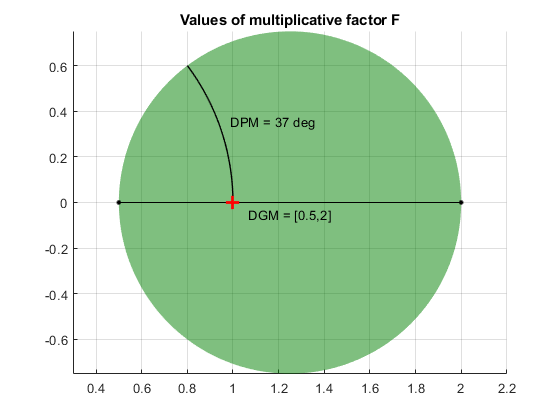In general, DGM or the corresponding DPM might capture larger ranges of variation than those you specify with the inputs GM and PM. The disk always captures at least the specified variations.

If GM is a column vector or matrix representing multiple target ranges of gain variation, DGM is a two-column matrix of the form [gmin1,gmax1; ...;gminN,gmaxN], where each row is a corresponding disk-based gain range.

Disk-based phase margin, returned as a two-element vector of the form [-pm,pm]. The amount of phase variation is determined by the geometry of the disk described by DGM (see Algorithms).

If PM is a column vector or matrix representing multiple target ranges of phase variation, DPM is a two-column matrix of the form [-pm1,pm1; ...;-pmN,pmN], where each row is a corresponding disk-based gain range.

Algorithms

umargin and diskmargin model gain and phase variations in an individual feedback channel as a frequency-dependent multiplicative factor F(s) multiplying the nominal open-loop response L(s), such that the perturbed response is L(s)F(s). The factor F(s) is parameterized by:

$F\left(s\right)=\frac{1+\alpha \left[\left(1-\sigma \right)/2\right]\delta \left(s\right)}{1-\alpha \left[\left(1+\sigma \right)/2\right]\delta \left(s\right)}.$

In this model,

• δ(s) is a gain-bounded dynamic uncertainty, normalized so that it always varies within the unit disk (||δ|| < 1).

• ɑ sets the amount of gain and phase variation modeled by F. For fixed σ, the parameter ɑ controls the size of the disk. For ɑ = 0, the multiplicative factor is 1, corresponding to the nominal L.

• σ, called the skew, biases the modeled uncertainty toward gain increase or gain decrease.

The factor F takes values in a disk centered on the real axis and containing the nominal value F = 1. The disk is characterized by its intercept DGM = [gmin,gmax] with the real axis. gmin < 1 and gmin > 1 are the minimum and maximum relative changes in gain modeled by F, at nominal phase. The phase uncertainty modeled by F is the range DPM = [-pm,pm] of phase values at the nominal gain (|F| = 1). For instance, in the following plot, the right side shows the disk F that intersects the real axis in the interval [0.71,1.4]. The left side shows that this disk models a gain variation of ±3 dB and a phase variation of ±19°.

DGM = [0.71,1.4]
F = umargin('F',DGM)
plot(F)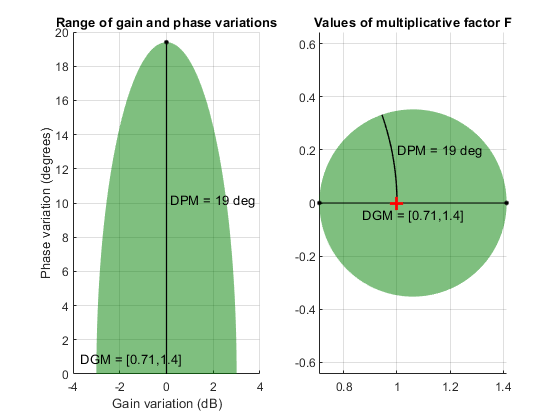getDGM converts the target gain and phase variations that you want to model into the disk-based gain-variation range DGM. This range fully characterizes the disk F. The corresponding phase range DPM is thus determined by DGM and the disk model.

For further details about the uncertainty model for gain and phase variations, see Stability Analysis Using Disk Margins.